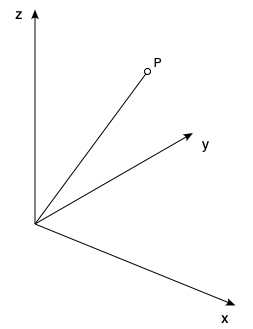# Uniform vector on sphere given rotation angles

By Paul Bourke
October 2020

The following is a quick note on the random rotation of a vector in 3D such that the distribution on the surface of a sphere is uniform. The coordinate system for this document is right handed as shown below, we will start with a vector along the z axis and wish to find rotation angles and axes that give the uniform distribution on the sphere of the resulting vector.A naive way may be to imagine just choosing three angles, one for each axis and each ranging from 0 to 2π. However this leads to a higher density of points along the equator.One might then realise that rotating by all three axes is not necessary, one can cover the sphere with just a rotation about 2 axes. For example, a rotation about the x axis by a uniformly distributed random angle between 0 and pi, and a rotation about the z axis by a uniformly distributed random angle from 0 to 2π However, this leads to a higher density of point at the two poles.The solution is to modify the first rotation about the x axis, specifically as follows, where u is uniform random distribution on the range 0 to 1.